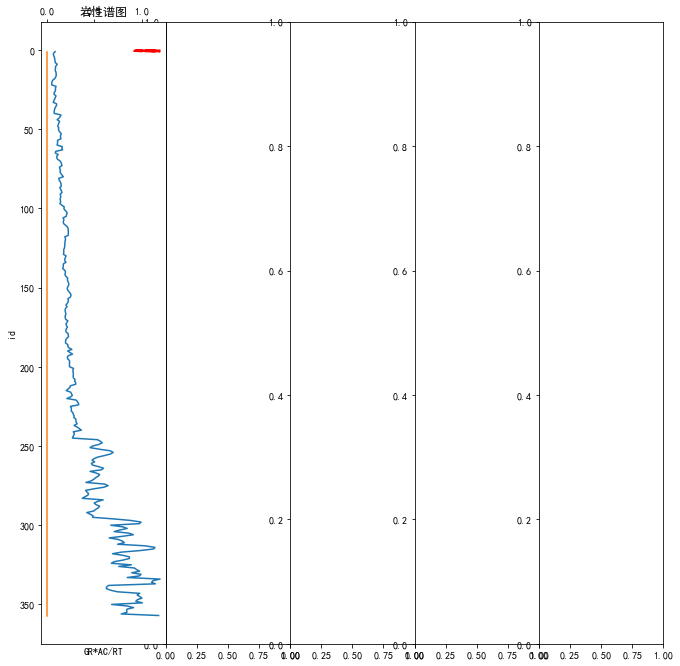（急！！！！）如何用python实现向左（y轴）填充颜色？

x、y不相关，只是连接呈曲线，想要曲线向左填充的话，怎么弄啊？

x = np.array(data['id'])
y = np.array(data['q'])
z = np.array(-data['q'])

plt.plot(x,y)
plt.plot(x,z)/201904/26/1556267295_134808.png)

plt.xlabel('x')
plt.ylabel('y')
plt.fill_between(x,y,where=(y<0.15),facecolor='yellow')

``````
``````1个回答